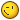# Mapping texture to a Graphics object

## Recommended Posts

Hi I was wondering if there's a way in Pixi to map a texture to a graphics object. I would like to some warping on and distortion to images but the only way I can see this happening if I can map a texture to the graphics object.

Thanks

##### Share on other sites

Its a problem: Mesh has Texture, but graphics has tesselation. However, some cases is solved with https://github.com/pixijs/pixi.js/pull/3842 , this is the branch: https://pixijs.download/dev-mesh-update/pixi.js

This is one of demos:

``````var app = new PIXI.Application();
document.body.appendChild(app.view);

var timeLine = 0;
var scale = 1.75;

var texture = PIXI.Texture.fromImage('required/assets/displacement_BG.jpg');
var strip = new PIXI.mesh.Plane(texture, 10, 10);
strip.scale.set(scale, scale);
var vertices = strip.vertices;
var calculatedVertices = strip.calculatedVertices;

strip.x = -30;
strip.y = -30;

var g = new PIXI.Graphics();
g.scale.set(scale, scale);
g.x = strip.x;
g.y = strip.y;

// start animating

timeLine += 0.05;
var index = 0;
for (var r = 0; r < 10; r++) {
for (var c = 0; c < 10; c++) {
var x = calculatedVertices[index * 2];
var y = calculatedVertices[index * 2 + 1];
vertices[index * 2] = x + Math.sin((index * 2) + timeLine) * 10;
vertices[index * 2 + 1] = y + Math.sin((index * 3) + timeLine) * 10;
index++;
}
}
renderVertices(vertices);
});

function renderVertices(vertices) {

g.clear();

g.lineStyle(2, 0xffc2c2, 0.5);
// g.moveTo(vertices, vertices);

var index = 0;
for (var r = 0; r < 10; r++) {
for (var c = 0; c < 10; c++) {
if (c === 0) {
g.moveTo(vertices[index * 2], vertices[index * 2 + 1]);
}else{
g.lineTo(vertices[index * 2], vertices[index * 2 + 1]);
}
index++
}
}

for (var i = 1; i < vertices.length; i++) {
g.beginFill(0xff0022);
g.drawCircle(vertices[i * 2], vertices[i * 2 + 1], 6);
g.endFill();
}
}
``````

Just open http://pixijs.github.io/examples/?v=dev-mesh-update#/basics/basic.js and copy-and-paste it there. Please try it in 10 minutes, we are building new version of it##### Share on other sites

Thank you so much, I'll be messing around with this for sure!

##### Share on other sites

The branch has new Mesh, Plane and Rope. " I would like to some warping on and distortion to images" - that's exactly what we are doing.

Switch Sprite to Plane and change vertices based on calculatedVertices, or switch Sprite to Rope and mess with points/calculatedPoints.

There are more demos in github link. I hope it will be merged into master soon##### Share on other sites

This takes Pixi to a whole other level. Thanks for the hard work.

## Join the conversation

You can post now and register later. If you have an account, sign in now to post with your account.
Note: Your post will require moderator approval before it will be visible.×   Pasted as rich text.   Paste as plain text instead

Only 75 emoji are allowed.